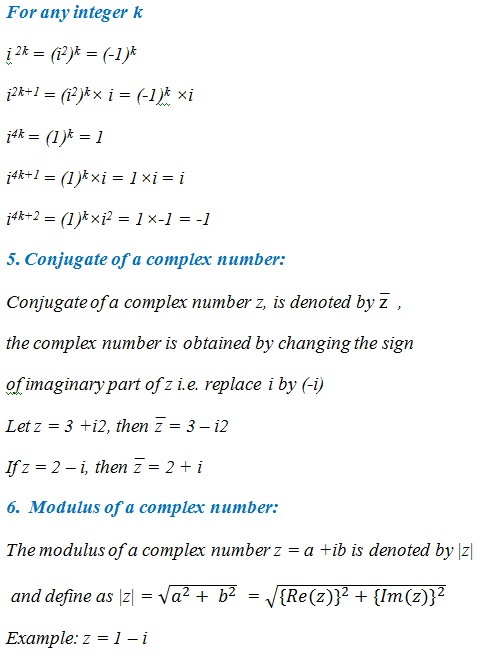Study Materials: CBSE Notes

Our CBSE Notes for Complex Number Class 11 maths 5. Complex Number and Quadratic Equations - CBSE Notes - Toppers Study is the best material for English Medium students cbse board and other state boards students.

Notes ⇒ Class 11th ⇒ Mathematics ⇒ 5. Complex Number and Quadratic Equations

# Complex Number Class 11 maths 5. Complex Number and Quadratic Equations - CBSE Notes - Toppers Study

Topper Study classes prepares CBSE Notes on practical base problems and comes out with the best result that helps the students and teachers as well as tutors and so many ecademic coaching classes that they need in practical life. Our CBSE Notes for Complex Number Class 11 maths 5. Complex Number and Quadratic Equations - CBSE Notes - Toppers Study is the best material for English Medium students cbse board and other state boards students.

## Complex Number Class 11 maths 5. Complex Number and Quadratic Equations - CBSE Notes - Toppers Study

CBSE board students who preparing for class 11 ncert solutions maths and Mathematics solved exercise chapter 5. Complex Number and Quadratic Equations available and this helps in upcoming exams 2023-2024.

### You can Find Mathematics solution Class 11 Chapter 5. Complex Number and Quadratic Equations

• All Chapter review quick revision notes for chapter 5. Complex Number and Quadratic Equations Class 11
• NCERT Solutions And Textual questions Answers Class 11 Mathematics
• Extra NCERT Book questions Answers Class 11 Mathematics
• Importatnt key points with additional Assignment and questions bank solved.

NCERT Solutions do not only help you to cover your syllabus but also will give to textual support in exams 2023-2024 to complete exercise maths class 11 chapter 5 in english medium. So revise and practice these all cbse study materials like class 11 maths chapter in english ncert book. Also ensure to repractice all syllabus within time or before board exams for ncert class 11 maths ex in english.

See all solutions for class 11 maths chapter 5 exercise 5 in english medium solved questions with answers.

### Complex Number class 11 Mathematics Chapter 5. Complex Number and Quadratic Equations

• Complex Number Class 11 Maths 5. Complex Number And Quadratic Equations - CBSE Notes - Toppers Study
• Class 11 Ncert Solutions
• Solution Chapter 5. Complex Number And Quadratic Equations Class 11
• Solutions Class 11
• Chapter 5. Complex Number And Quadratic Equations Complex Number Class 11

## Complex Number Class 11 maths 5. Complex Number and Quadratic Equations - CBSE Notes - Toppers Study

### 4. Power of i (iota)Important Study materials for classes 06, 07, 08,09,10, 11 and 12. Like CBSE Notes, Notes for Science, Notes for maths, Notes for Social Science, Notes for Accountancy, Notes for Economics, Notes for political Science, Noes for History, Notes For Bussiness Study, Physical Educations, Sample Papers, Test Papers, Mock Test Papers, Support Materials and Books.Mathematics Class - 11th

NCERT Maths book for CBSE Students.

books

## Study Materials List:

##### Notes ⇒ Class 11th ⇒ Mathematics
1. Sets
2. Relations and Functions
3. Trigonometric Functions
4. Principle of Mathematical Induction
5. Complex Number and Quadratic Equations
6. Linear Inequalities
7. Permutations and Combinations
8. Binomial Theorem
9. Sequences and Series
10. Straight Lines
11. Conic Sections
12. Introduction to Three Dimensional Geometry
13. Limits and Derivatives
14. Mathematical Reasoning
15. Statistics
16. Probability

New Books